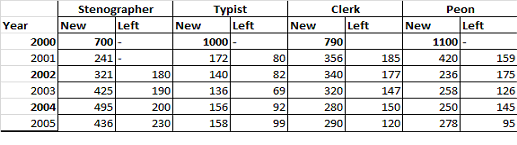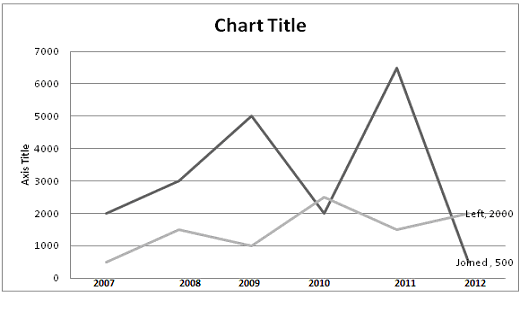# IBPS Quantitative Aptitude model test paper - 6

Home > Aptitude test > Quantitative model test - 6

## IBPS Quantitative Aptitude model test paper - 6

Directions (Q.1-5) What value should come in place of the question mark (?) in the following question?

Q1. √10000 + √9801 = ?

A. 1
B. 199
C. 89
D. 19

Q2. 981 ÷ 9 – 121 + 512 = ?

A. 1000
B. 50
C. 500
D. 50.75

Q3. 1011 - ? = 0.0105 x 0.99 x 0.14 .

A. 1010.999
B. 1.999
C.1011.011
D. 1000

Q4. 33.33% of 3333 - 11.11% of 1111 + 12.5432 = ?

A. 1000
B. 100
C. 9999
D. 1254

Q5. 21/35 of 18/54 of 21/84 of 100 =?

A. 150
B. 50
C. 5
D. 55

Q6. 18 boys can do a piece of work in 30 days. How many boys are required to do a piece of work in 15 days?

A. 38
B. 36
C. 30
D. 40

Q7. Find the average of following set of numbers?

89, 47, 85, 25,47, 36,96 .

A. 60.71
B. 64.74
C. 48.80
D. 85.50

Q8. The average of 5 numbers is 51.4 . And the average of first two number is 30.5 and the average of last two number is 62. Find the middle number?

A. 70
B. 82
C. 88
D. None of these

Q9. What will come in place of question mark (?) in the following number series?

93 68 48 ? 23 18

A. 28
B. 35
C. 42
D. 33

Q10. Harsh started a business by investing Rs. 45000. After 8 months, Uday joined with sum of Rs. 52000. At the end of year the profit is Rs. 56165, what is the share of Uday in

profit?

A. 22480
B. 21500
C.15618
D. 11482

Q11. The following questions are accompanied three statement a, b & c. You have to determine which statement(s) is /are necessary sufficient to answer the question?
What is the speed of the bike ?

a. The bike crosses a pole in 13 seconds.
b. The bike crosses a platform of length ‘x’ m in ‘y’ minutes.
c. The bike crosses another bike running in the same direction in 45 minutes.

A. Only a .
B. Only b.
C. Only a & b.
D. Only c.
E. None of these

ANSWER: C. Only a & b.

Directions (Q. 12-16). The following table shows the number of new employees added to different categories of employees in a Company and also the number of employees from these categories

who left the company every year since the foundation of the company in 2000.Q12. What was the total number of Typist working in the company in the year 2003?

A. 917
B. 1217
C. 67
D. None of these

Q13. What is the pooled average of the total number of employees of all categories in the year 2002?

A. 105.75
B. 1194.5
C. 1309.50
D. 1445

Q14. What is the difference between the total number of Clerks added to the company and the total number of Typist added to the company during the years 2002 to 2005?

A. 800
B. 226
C. 640
D. 128

Q15. For which of the following categories the percentage increase in the number of employees working in the company from 2000 to 2005 was the maximum ?

A. Typist
B. Clerk
C. Peon
D. Stenographer

Q16. During the period 2000 to 2005 , the total number of Stenographers who left the company is what percent of the total number of Stenographer who joined the company ?

A. 30
B. 20
C. 45
D. 65

Directions (Q. 17-18). In the following questions two equations numbered I and II are given. You have to solve both the equations and give answer

1) If a> b
2) If a>= b
3) If a < b
4) If a=5) If a= b or the relationship cannot be established

Q17. I. 3a -2b - 10 = 0
II.5a -6b – 6 = 0

A. Only 1 follows
B. Only 2 follows
C. Only 4 follows
D. Only 5 follows.

Q18. I. a2 = -9a -18.
II.b2 = 13b – 40

A. Only 1 follows
B. Only 2 follows
C. Only 3 follows
D. Only 4 follows.

Q19. One card is drawn by Anuj at random from a pack of playing cards. What is the probability that the card drawn is a face card (Jack, Queen and King only)?

A. 3/52
B. 4/52
C. 11/26
D. None of these.

Q20. Find the compound interest on a sum of Rs. 50,000 after 3 years@12% per annum?

A. 22000
B. 70246.4
C. 20246.4
D. 50000

Directions (Q. 21-25) Study the following line graph which gives the number of employees who joined and left the company in the beginning of the year for five years, from 2007 to 2012.
Initial Strength of the company in 2006 = 3000Q21. The strength of the company increased/decreased from 2007 to 2008 by approximately what percent ?

A. 60%
B. 33%
C. 45%
D. 15%

Q22. The number of employees working in the company during 2009 was :

A. 6000
B. 9500
C. 10000
D. 6000

Q23. During which of the following pairs of years, the strength of the employee was same ?

A. 2007 and 2008
B. 2009 and 2011
C. 2008 and 2012
D. None of these

Q24. The number of employees working in the company in 2010 was what percent of the number of employees working in the company in 2012?

A. 25%
B. 55%
C. 75%
D. None of these

Q25. Among the given years, the largest number of the employees joined the company in the year?

A. 2009
B. 2010
C. 2011
D. 2008